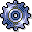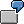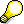Plan Accrual CalculationUse

Plan accrual calculation lets you plan accrual costs: Accrual costs are subdivided as follows:

Costs that have no equivalent in Financial Accounting (for example, managerial income or imputed rents)

• Valuation differences

Costs that have a different equivalent in FI (for example, vacation bonuses or insurance)

Payroll fringe costs such as bonuses, which can generally be calculated as a percentage rate of the wage or salary costs, are not usually calculated and planned on every individual cost center. You can calculate these using the plan accrual methods.The vacation bonus is accrued on the basis of the plan labor costs using an a overhead rate of 10%. The system debits all cost centers on which labor costs are planned.The SAP R/3 System takes into account the activity-dependent plan costs during the calculation of accrual amounts using the percentage method, which assigns a percentage rate to the fixed and variable portions of the overall costs.You have planned a total of \$200,000 salary costs on cost center 4310. This includes fixed costs of \$60,000. The overhead rate for the vacation bonus is 30%. The R/3 System calculates the following accrual amounts:

 Total Fixed 200,000 * 0.3 = 60,000 60,000 * 0.3 = 18,000